# Electrical Vehicle Route Planning on Google Map with Reinforcement Learning

More datail please find the `Paper.pdf` experiment section. This work is aim to take battery, motor, traffic time, and other factor into account for generating better route (minimize the energy consumption) for the vehicle under certain condition.
The other related personal project can be found at this link: https://angel.co/dung-yi-chao. Please read carefully through this page before you start running the program. There are many parameters or condition need to be modified in order to fit your environment.

## Library Requirements with Python 3.6.2:

(1) Tensorflow (CPU) (2) numpy (3) pandas (4) haversine (5) time (6) random (7) math (8) requests (9) urllib

## Set Up Google Map API

Google Map API is now requiring every search URL contains user's key. Please refer to this link: https://cloud.google.com/maps-platform/ . Enter your billing information, it will not charge you at this moment because you got \$200 free search credit. If you exceed the certain amount of search, then you will be charged. You only need the Maps option.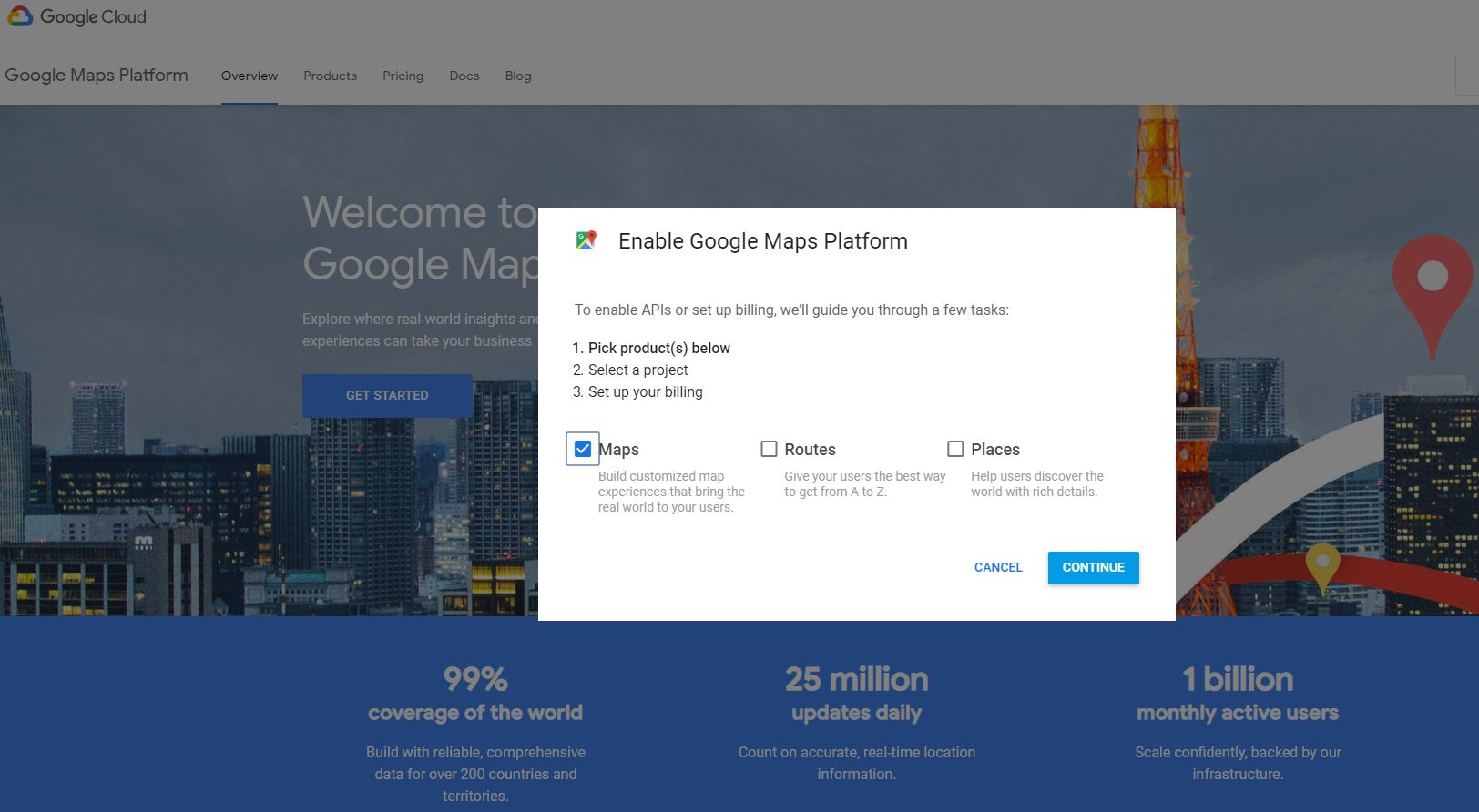You will get a key for example `AZuesYuds12_dsakd23456sdeHf`.Then the searching URL of (latitude, Longitude) = (40.468254,-86.980963) will become: https://maps.googleapis.com/maps/api/geocode/json?address=40.468254%2C-86.980963&key=AZuesYuds12_dsakd23456sdeHf

The result of the URL (with your correct key) will be like the following figure.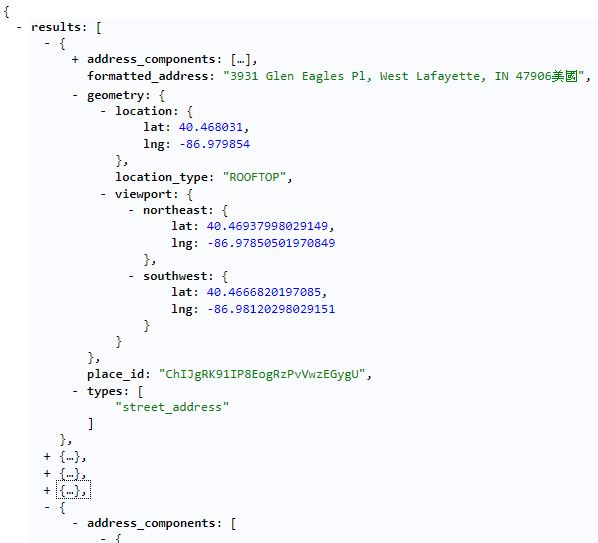There are three places in the `Environment.py` where you need to add the key like the figure below. I've put these fake key in the code for you. What you need to do is replace these fake key with your own key.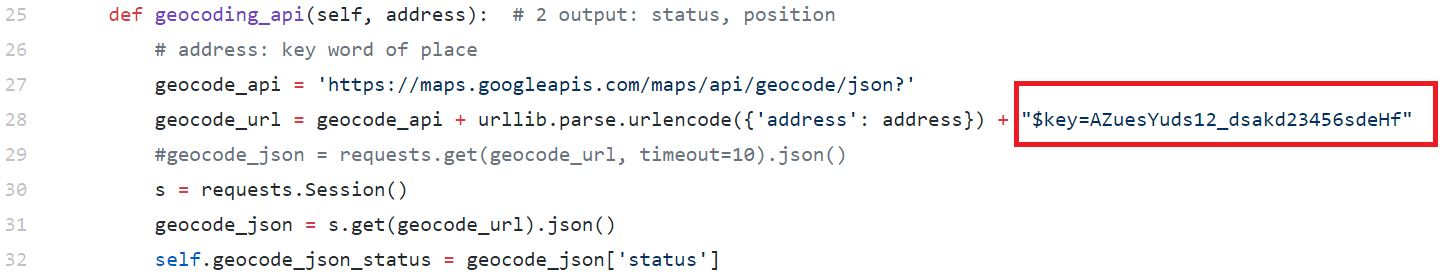You can learn how to interact with google map api (geocode/elevation/directions) correctly in `Environment.py`.

Notice that my research and result was conducted in 2017 in Purdue ME linux server. During that time, I'm free to do the search without paying any fee (Sometimes my search would stop because Google map server would block my ip because I've done too much query. Wait for 5 to 10 min or sometimes even longer such as 1 to 2 hours, and the server would unlock my ip.) I'm sure Google Map server is not going to block your ip any more because they will charge your credit card directly.

## How can you get started

Download all the python file as following: `main.py`, `Environment.py`, `DoubleDQN.py`, `battery.py`, `motor.py`

• enter your start position in `main.py`: name of position or geocode (lat, lng)
• enter your destination position in `main.py`: name of position or geocode (lat, lng)
• enter the length of each step (step_length) in `main.py` line 78, higher but less accurate (ex: 1000m takes less time to train compare to 100m)
• enter how many episode you want to train in `main.py`
• There are many file and folder path name need to be changed in `main.py` to fit your condition.
• Make sure that you can access to the internet and google map during the training process. Notice that your server will be blocked if your access to google map API exceed the limit in 24 hour and your program will be stucked. In the `main.py` we have implemented a mechanism to make your program sleep for a while when you over query the data. If you can get the full access to the Google map API, then you can remove the `sleep` command and make the learning process faster. (This rule might be changed with new rule adopted by Google Map Server. If so, please disable all the sleep command ~ edit on April, 2019)
• If you never train any model yet, then you need to make `load_model == False` in the line 141 of `main.py` .
• Run file `main.py`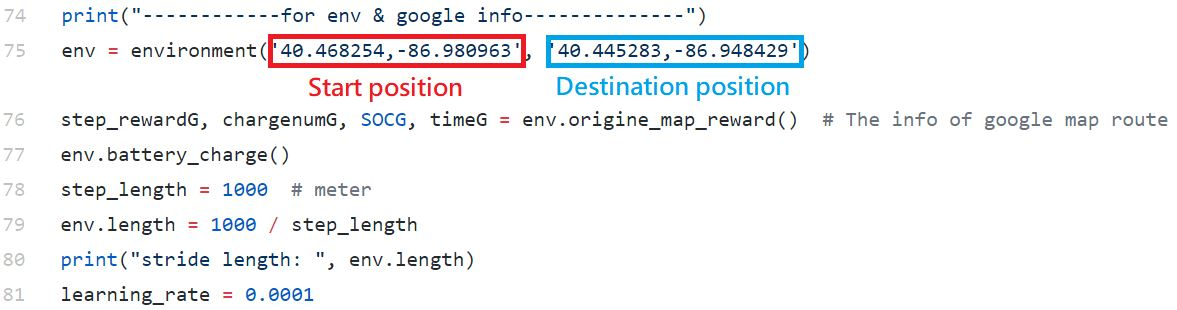## How can you be creative

• You can model a real battery system which can include the battery degradation, SOC and other factor to make the whole system more like a real vehicle. The model can be implement in the python file: `battery.py`. In the original python file, we only model the battery in linear manner.
• You can model a real motor system which can include the motor fatigue, heat condition and other factor to simulate the real motor. Your model can be implement in the python file: `motor.py`. In the original python file, we only model the motor with ideal manner. You can find out the real factor between input energy and output energy in the motor and apply it in the code.
• You can have a more complicated neural network architecture to deal with larger map boundary and implement in the file: `DoubleDQN.py`. The function of this python file is to get input from the environment (such as state) and output an action (it can be Q values)
• You can implement other learning algorithm other than Double-DQN in the file: `main.py`. This file will creat two .csv files , one record the training environment parameter names `train_para.csv` and the other one names `result.csv` will record the training data such as reward, duration, failed steps, step history and so on. The learning model and checkpoint is also saved in a folder names `model`.
• You can add more action choices in the function of `step` of file: `Environment.py` such as heading southwest or northeast. In the origine python file, we only implement 4 action: north, east, south and west. It is also possible to change the way you calculate the energy consumption and add the concept of regenerative brake.

## How does this work

### (1) Algorithm: Double-DQN

The basic idea of the reinforcement learning is to make the agent learn from the action it takes and the feedback it gets. When the agent encounter a current state, it then decide what to do next and give an action. By executing this action, the agent will enter the next state. This routine will continue untill the agent reach the destination or terminate by the limit of the number of actions it should take.

We initialize two identical network, the first one is the Q-network which the agent determines the action at the current state. The second one is the Target-network which acts as a target for Q-network to achieve. We only do backpropagation and update the weights through AdamOptimizer with learning rate 0.0001 in the Q-network for every steps and then copy the weights in Q-network to Target-network for every N steps (5 steps is implemented in the original python file).

Our learning agent is an electric vehicle and navigating on the Google map environment by choosing different action (north, east, south, west). The action can be determined by the Double-DQN or by random. During the learning process, the agent will first navigate on the map randomly to explore the map, but we will gradually reduce the portion of choosing action randomly but adopt the action with highest q value provided by the Double-DQN model. We save current state, action, current reward, next state, reach end or not in a tuple and store every tuple in a replay buffer which acts like a brain that we store all the memory in. When we need to update the Q-network weights, we will pick Nb number of tuples randomly and uniformly from the replay buffer. Here comes the action of Target-network, it will take the next state of a tuple as an input and output a vector of q value corresponds to each action choice. We only pick up the value among this vector of q value which corresponds to the action that gives us the highest value when inputing the next state into the Q-network, and then we compute the target y by adding this value with factor γ to the current reward. We implement γ as 0.9 and Nb as 32 in orginal case.

Given a tuple from the replay buffer, the loss is computed by subtracting the q value which is calculated by inputing the current state into the Q-network and pick the value corresponding to the action from y. We then add up all the loss (we have 32 losses in our case) and use it to update the Q-network's weights.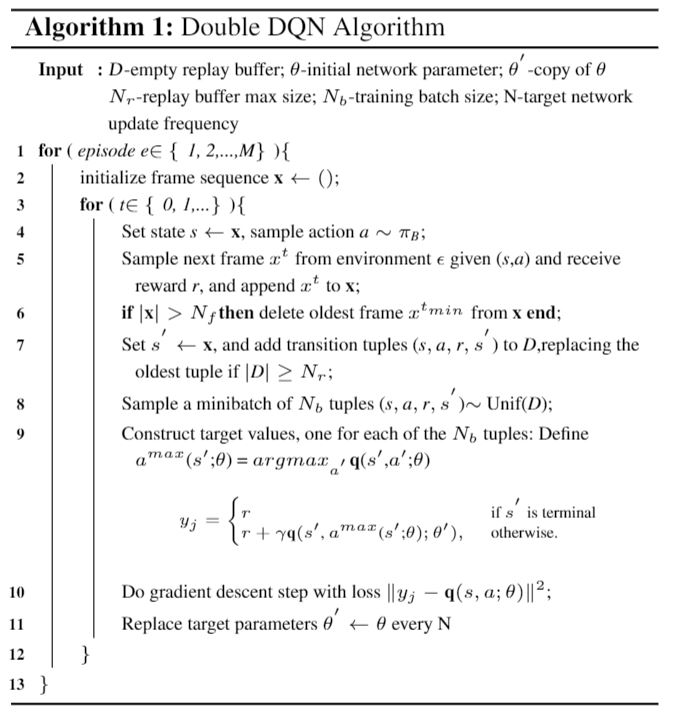### (2) Learning Environment

We make the map like a grid map for the learning agent to navigate on which is shown in figure(a). Strictly speaking, each grid in the grid map is not a rectangle. This phenomenon is caused by the sphere geometry and our restriction on the length of the stride which is demonstrated in figure (b). The reason for restricting the length of stride to certain meter is to bound the inaccuracy within the given length.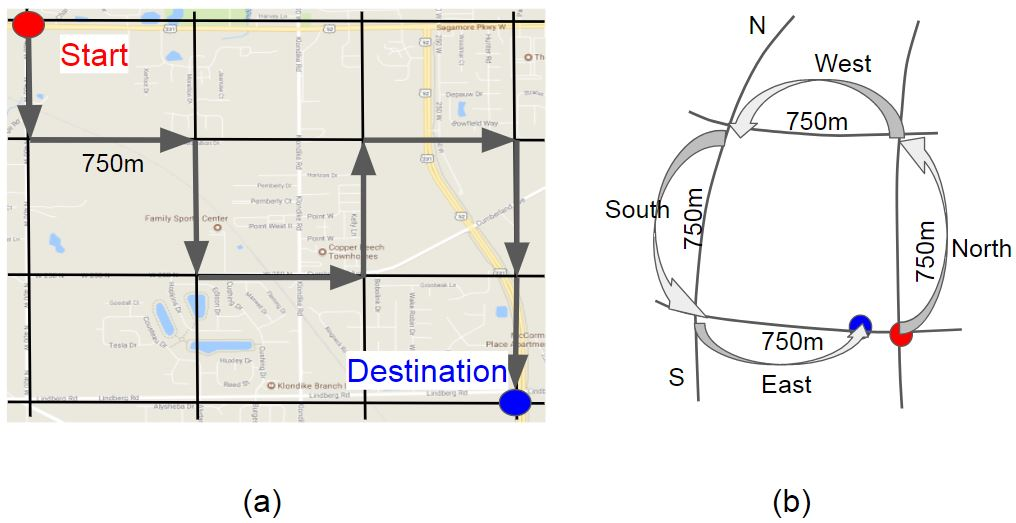### (3) Interact with Google map API

There are 4 action in the action set, north, east, south, and west. If your current position is (latitude, Longitude) = (40.468254,-86.980963) and you want to take the action of heading north, then your next position will become (latitude, Longitude) = (40.468254 + value1, -86.980963). The value1 need to be calculated from the step_length. If you want to take the action of heading east, then your next position will become (latitude, Longitude) = (40.468254, -86.980963 + value2). The value2 need to be calculated from the step_length as well the current latitude (value2 will be different based on the agent current latitude, but all the value of value2 should reflect the step_length). The new calcultated position is not accessible guarantee from the current position, if the direction is not accessible (for example, lake or forest), Google server might return your direction query a status which is not 'ok'.

Assume that the agent is at current position denoted by A and heading south to the next position denoted by B. Apparently, the route provided by the Directions API is the highway 395 and the distance is longer than 1000m. We can get the navigating instruction list with the form: { geocode of A, duration from A to 1, distance from A to 1, geocode of 1 }, {geocode of 1, duration from 1 to 2, distance from 1 to 2, geocode of 2 }.... where A, 1, 2 ,3 , B are shown in figure 6 after we input the geocode of A and the geocode of B into the Directions API. The number of instruction is based on the Directions API and there are four instructions in our case of following figure from A to B. We use each of the geocode in the navigating instruction list to get the height of each position from the Elevation API and compute the elevation within each instruction.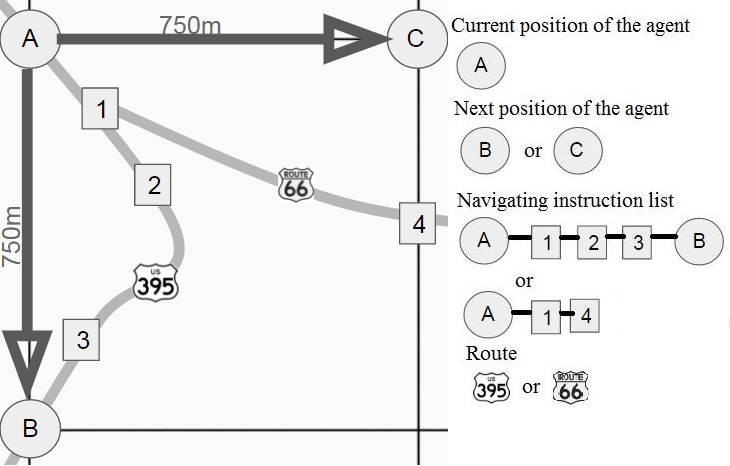### (4) Energy Computation

We compute the energy by the following flow chart which the symbol is corresponding to the previous figure.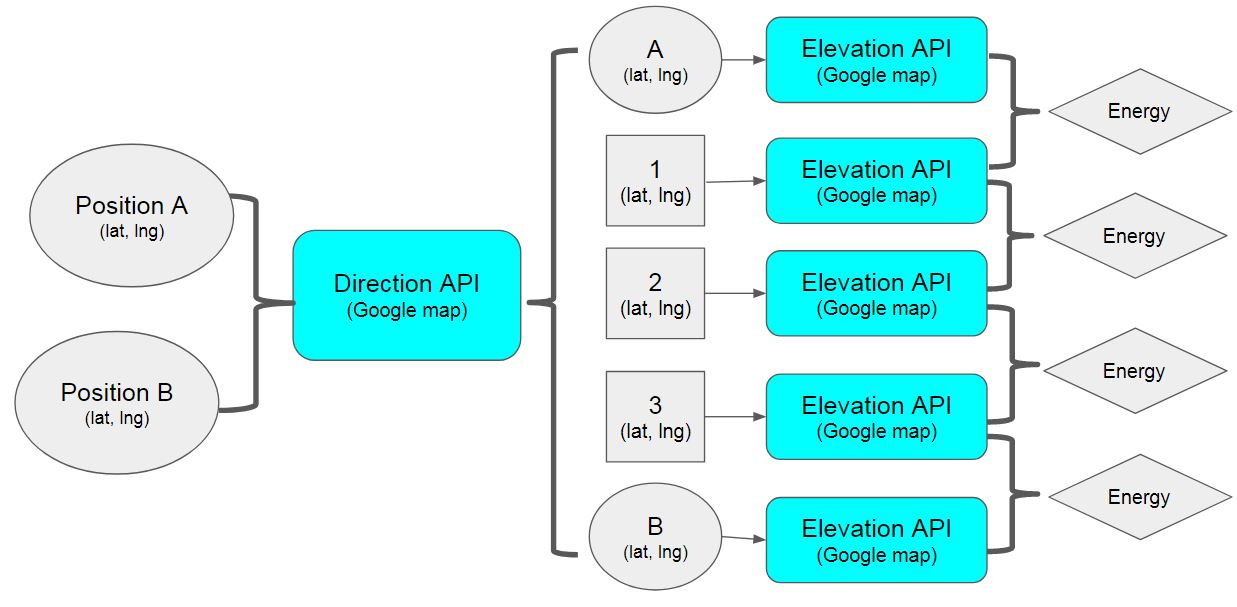The following graph shows how to compute the energy for a vehicle to travel uphill (can also be applied on a flat road)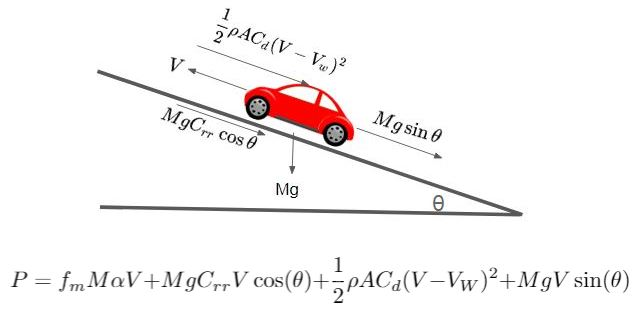But noticed that we only compute the elevation between the two position shown in the following graph. To increase the accuracy, you should minimize the distance between these two position (this will increase the computation time).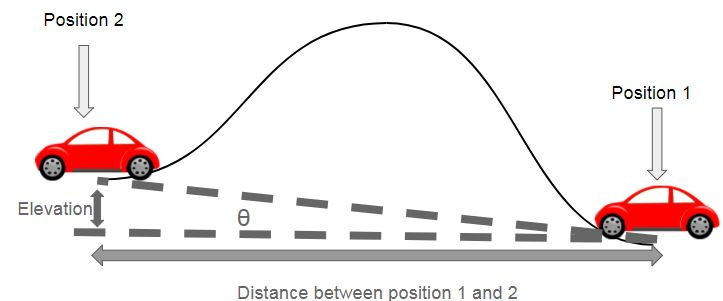### (5) Battery

In the experiment, the battery performance will not affect the training process. The battery is able to carry totally 50000Wh of energy which is a standard offering by electrical vehicle manufacture, Tesla. In electrochemistry, it is recommended to use the the state of charge (SOC) from 90% ~ 20% of a battery to improve it's life which we implement in our case. The SOC is calculated by the ratio of the current energy to the total energy. We will not take the battery degradation into the experiment. Further work can take the real factor on battery performance into consideration as part of the training process. For this experiment, we only demonstrate how much energy consumed and how many times the battery need to be charged in an ideal condition.

### (6) Reward arrangement

The fundamental concept of defining the reward is based on the energy consumption in one stride from the current position to the next position, for example, from A to B shown in the figure 6. The energy is calculated by the method provided previous section. We then divide the energy by 10000 and times -1. In order to minimize the number of total steps during training, we add -0.1 to each transition if the next position is reachable. In other words, the reward r for taking any reachable step will be r = -0.1 - (energy consumption / 10000). If the next position is unreachable such as a lake or a river, r = -1 and the agent stay at the same current position and take the other action. If the distance of the next position and the destination position is less than the length of the predefined displacement (1000m in the figure 6), the reward r for taking this action will become r = +1 - (energy consumption from the current position to the next position / 10000) - (energy consumption from the next position to the destination position / 10000). Noticed here +1 appears in the reward because of the success of this action which lead the agent to the destination.

• Black: Unreachable or out of boundary
• Red:: Start position
• Blue: Destination position
• Grean: Reachable position to reachable position where is not within the range of one stride of the destination
• White: Reachable position to the position where is in the one stride length of range from the destination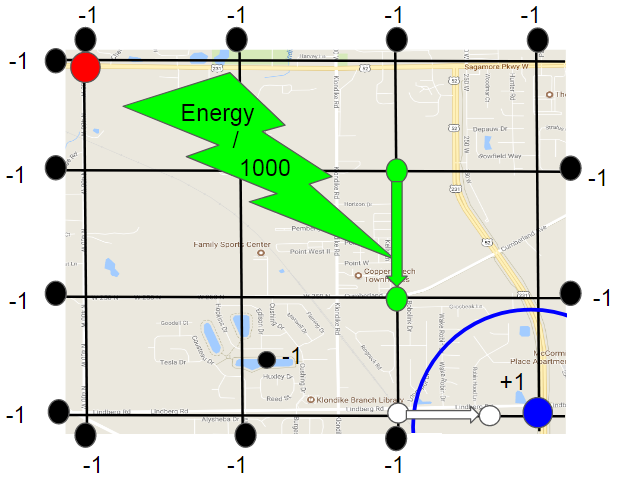## Result

The agent is being trained from the start position (geocode:40.4682572, -86.9803475) and the destination (geocode:40.445283, -86.948429) with stride length 750m. Steps more than 64 steps within an episode will be regarded as failed. Noticed that during the training, Google map api often blocked our server and we are forced to end the training process and resume the model from the interrupted episode (red line). This problem will lead to the empty replay buffer where we choose sample uniformly at random to compute the loss and updtate the weights during learning. As a result, we will need to resume the model and start choosing action randomly to refill the replay buffer and gradually decrease the portion of random action.

The blue line in figure 9 shows the energy consumed by the agent. The agent is able to find out a way to minimize the energy consumption after 600 episodes. The oscillation in the first 600 episodes is caused by highly random action (green line) and inaccurate Q value provided by the Q-network. While the loss of inaccurate Q value is minimized, the oscillation is mitigated and the energy consumption become less and stable. The minimum energy that the agent can achieve with random action is 1327(J) and the corresponding time is 659 seconds where the energy and time of the route provided by Google map are 1489(J) and 315 seconds.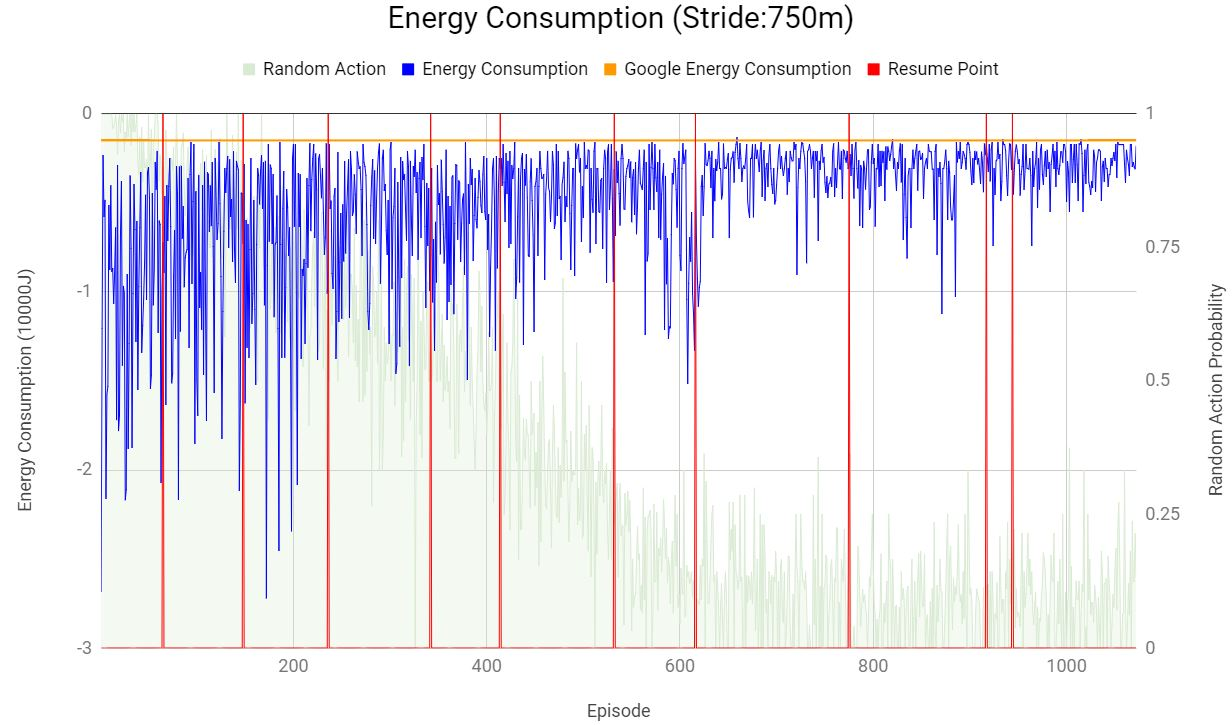The lower figure is the result of energy consumption with stride length of 1000m. Steps more than 36 steps within an episode will be regarded as failed. The minimum energy that the agent can achieve with random action is 1951(J) and the corresponding time is 946 seconds. We didn't make this experiment end at the same point as the previous one because we just want to demonstrate the trend that with lower stride length we can achieve better result but more computation time.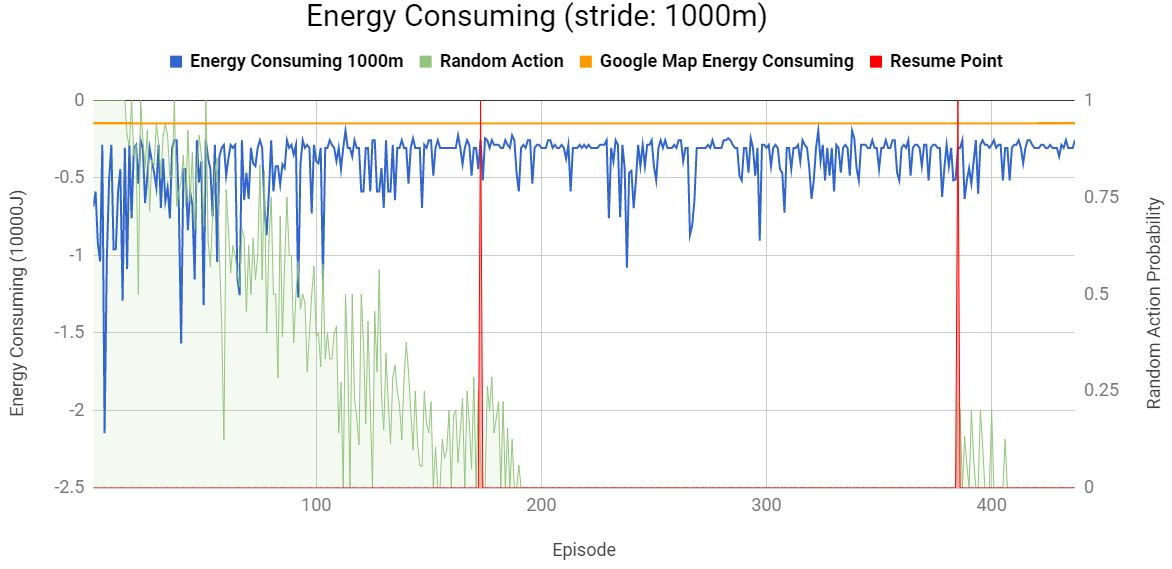This work is only an experiment of my concept and was a course project of ECE570 Artificial Intelligence in 2017 Fall. The parameters and model are not optimized. If you can take larger map into training (Of course you will be charged a lot by Google), and improve the models, I'm sure you can get a better result.

Currently, the input is merely the latitude and longitude. I think there is a serious problem of negative value of latitude and longitude which I didn't think of when I implemented the project. Reader can make an improvement on this.

We did not validate our model in any unseen map, and here comes to the basic question, can this model be used in an unseen world? I don't know.

Is it a good idea to use latitude and longitude as input? I doubt it, after I done this project. What will happen if there are some new road being constructed? Are we going to train the model again? Maybe feeding the screenshot of the map as input will be a good idea and the idea will be similar as Value Iteration Network (link: https://arxiv.org/abs/1602.02867 ) which I reviewed it in the Paper survey section.

## Credit

I learn a lot from Arthur Juliani's tutorial website which implement reinforcement learning algorithm with tensorflow. I also reference part of his code and modified it. (link: https://medium.com/emergent-future/simple-reinforcement-learning-with-tensorflow-part-0-q-learning-with-tables-and-neural-networks-d195264329d0)

I also encountered problem when initializing two networks (using the same model) of tensorflow. The following link solve the problem (link: https://stackoverflow.com/questions/41577384/variable-scope-issue-in-tensorflow). The following figure is where I implemented this concept.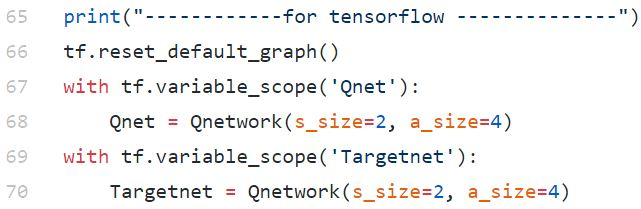Get A Weekly Email With Trending Projects For These Topics
No Spam. Unsubscribe easily at any time.
Python (1,140,683
Reinforcement Learning (3,847